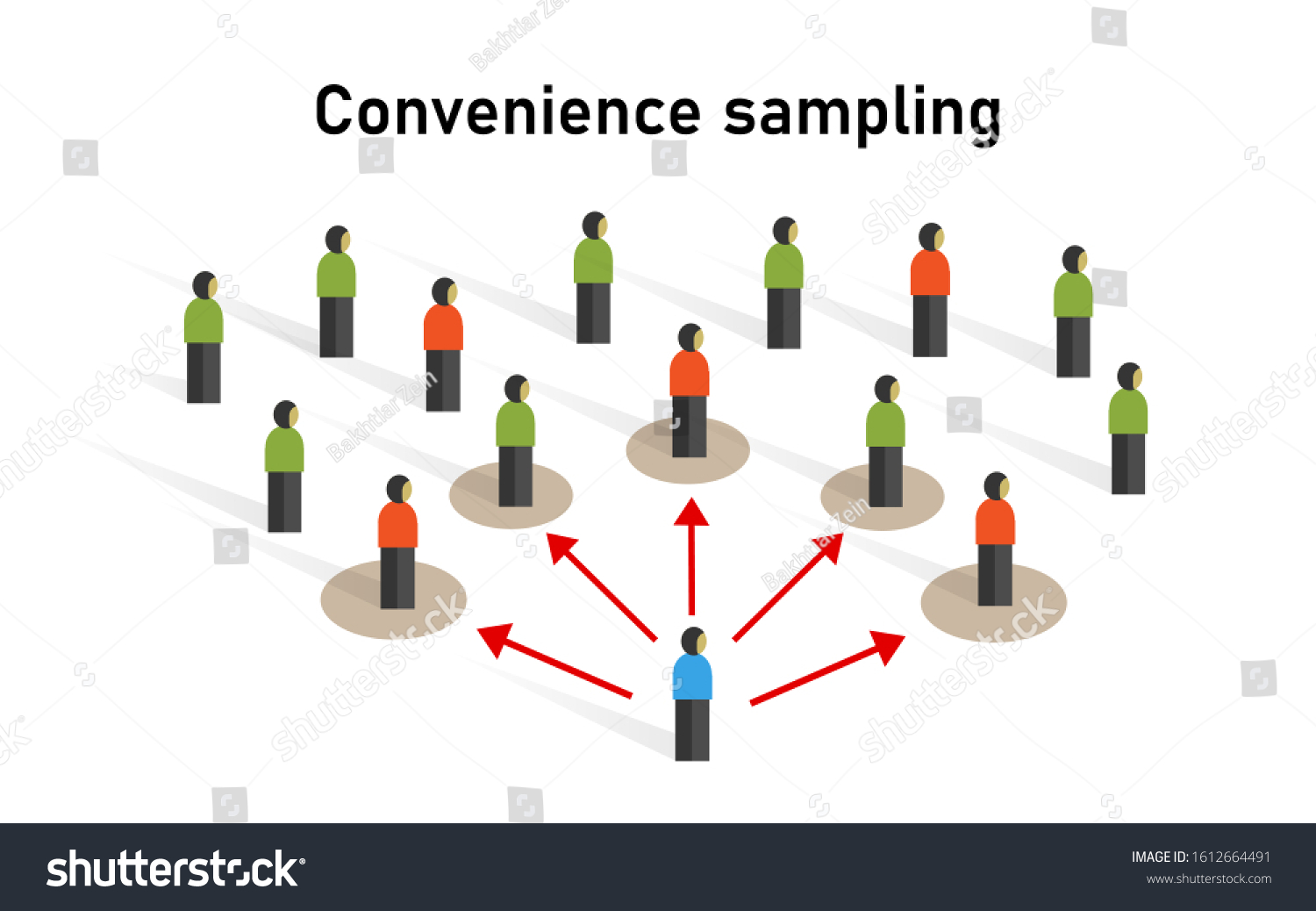### Statistical inference and sampling assumptionsfrom Lars SyllReal probability samples have two great benefits: (i) they allow unbiased extrapolation from the sample; (ii) with data internal to the sample, it is possible to estimate how much results are likely to change if another sample is taken. These benefits, of course, have a price: drawing probability samples is hard work. An investigator who assumes that a convenience sample is like a random sample seeks to obtain the benefits without the costs—just on the basis of assumptions. If scrutinized, few convenience samples would pass muster as the equivalent of probability samples. Indeed, probability sampling is a technique whose use is justified because it is so unlikely that social processes will generate representative samples. Decades of survey research have demonstrated that when a probability sample is desired, probability sampling must be done. Assumptions do not suffice. Hence, our first recommendation for research practice: whenever possible, use probability sampling.

If the data-generation mechanism is unexamined, statistical inference with convenience samples risks substantial error. Bias is to be expected and independence is problematic. When independence is lacking, the p-values produced by conventional formulas can be grossly misleading. In general, we think that reported p-values will be too small; in the social world, proximity seems to breed similarity. Thus, many research results are held to be statistically significant when they are the mere product of chance variation.

Richard Berk & David Freedman

In econometrics one often gets the feeling that many of its practitioners think of it as a kind of automatic inferential machine: input data and out comes casual knowledge. This is like pulling a rabbit from a hat. Great — but first you have to put the rabbit in the hat. And this is where assumptions come into the picture.

The assumption of imaginary ‘super populations’ is one of many dubious assumptions used in modern econometrics and statistical analyses to handle uncertainty. As social scientists — and economists — we have to confront the all-important question of how to handle uncertainty and randomness. Should we define randomness with probability? If we do, we have to accept that to speak of randomness we also have to presuppose the existence of nomological probability machines, since probabilities cannot be spoken of – and actually, to be strict, do not at all exist – without specifying such system-contexts. Accepting a domain of probability theory and sample space of infinite populations also implies that judgments are made on the basis of observations that are actually never made!

Infinitely repeated trials or samplings never take place in the real world. So that cannot be a sound inductive basis for a science with aspirations of explaining real-world socio-economic processes, structures or events. It’s not tenable.

And as if this wasn’t enough, one could — as we’ve seen — also seriously wonder what kind of ‘populations’ these statistical and econometric models ultimately are based on. Why should we as social scientists — and not as pure mathematicians working with formal-axiomatic systems without the urge to confront our models with real target systems — unquestioningly accept models based on concepts like the ‘infinite super populations’ used in e.g. the ‘potential outcome’ framework that has become so popular lately in social sciences?

One could, of course, treat observational or experimental data as random samples from real populations. I have no problem with that (although it has to be noted that most ‘natural experiments’ are not based on random sampling from some underlying population — which, of course, means that the effect-estimators, strictly seen, only are unbiased for the specific groups studied). But probabilistic econometrics does not content itself with that kind of populations. Instead, it creates imaginary populations of ‘parallel universes’ and assume that our data are random samples from that kind of  ‘infinite super populations.’

But this is actually nothing else but hand-waving! And it is inadequate for real science. As David Freedman writes:With this approach, the investigator does not explicitly define a population that could in principle be studied, with unlimited resources of time and money. The investigator merely assumes that such a population exists in some ill-defined sense. And there is a further assumption, that the data set being analyzed can be treated as if it were based on a random sample from the assumed population. These are convenient fictions … Nevertheless, reliance on imaginary populations is widespread. Indeed regression models are commonly used to analyze convenience samples … The rhetoric of imaginary populations is seductive because it seems to free the investigator from the necessity of understanding how data were generated.

In social sciences — including economics — it’s always wise to ponder C. S. Peirce’s remark that universes are not as common as peanuts …